# What is PCD ? How to Calculate PCD of Flange?

The PCD of a flange is one of the critical dimensions.
PCD is called as Pitch Circle Diameter and  usually measured in millimeters. It is usually used to measure the distance between the two opposite holes. If there are certain number of holes are in a circle and divided equally then we say the diameter of the circle as pitch circle diameter.

How to Calculate the PCD of Flange ?

To calculate the division of the PCD, multiply the diameter of the pitch circle and the given factor in the table. If 10 holes to be divided in a 100 mm pitch circle then the division, a = pcd x 0.309 = 100 x 0.309 = 30.90 mm. Here, PCD means Pitch circle diameter and so diameter 100 is taken as PCD.

Here are the Formulas to Calculate the PCD of Flange according to there Holes

 No. of holes in the Pitch Circle Diagram Formula 3 holesa = pcd x 0.866 4 holes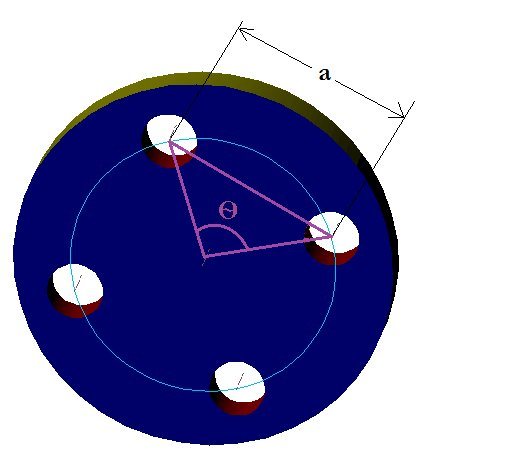a = pcd x 0.7071 5 holes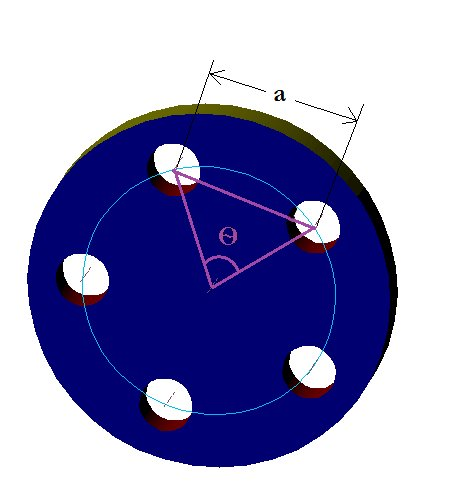a = pcd x 0.5878 6 holes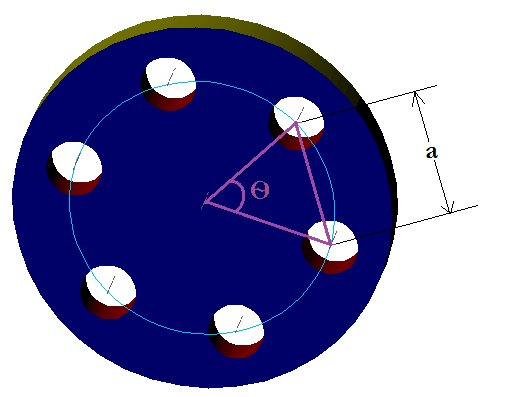a = pcd x 0.5 7 holes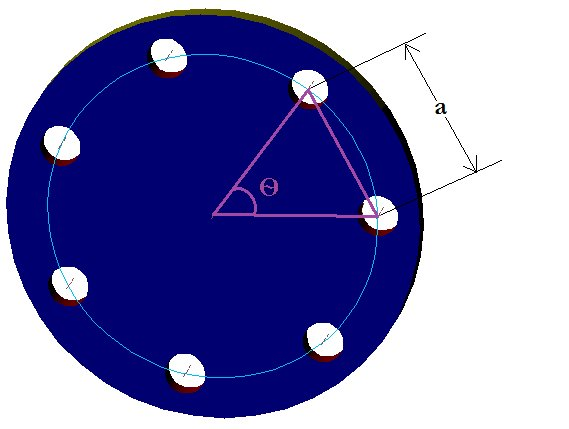a = pcd x 0.4339 8 holesa = pcd x 0.3827 9 holes a = pcd x 0.342 10 holes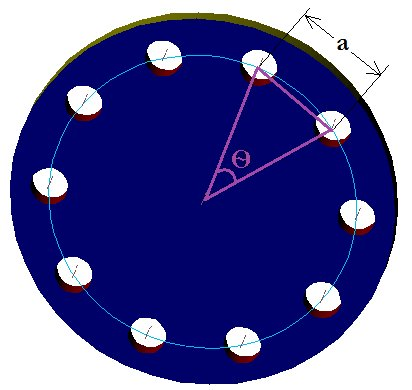a = pcd x 0.309

For Example :-

1.